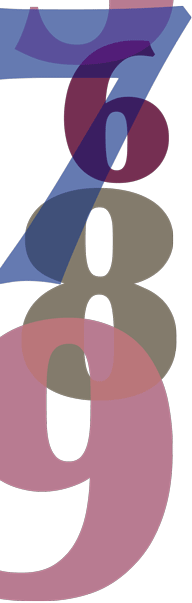# Year 7

## Number and Algebra

### Multiplication and division of decimals

Decimals are used widely. Our money is based on a decimal system. Common measurements such as the heights of students in a class, the price of petrol or the amount of electricity used per quarter in your home are usually expressed using decimals.

We can represent decimals as points on the number line by repeatedly subdividing intervals into tenths. A decimal number gives the 'address' of a point on the number line—the digits give successively more accurate information and together locate a point. This is an extension of the concept of place value met earlier. Whole numbers are written as strings of digits that correspond to the 'places'—ones, ten, hundreds, and so on. Positive numbers less than one can be written with the same notation with additional 'places'—tenths, hundredths, thousandths, and so on.

Despite their common use today, decimals were not used widely until the early seventeenth century. Now, decimals are used extensively and almost exclusively in commerce and science.

Because decimals are used so widely, it is very important that the inverse operations of multiplication and division are mastered by all students.